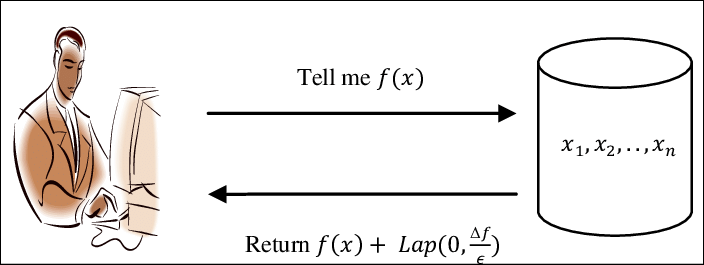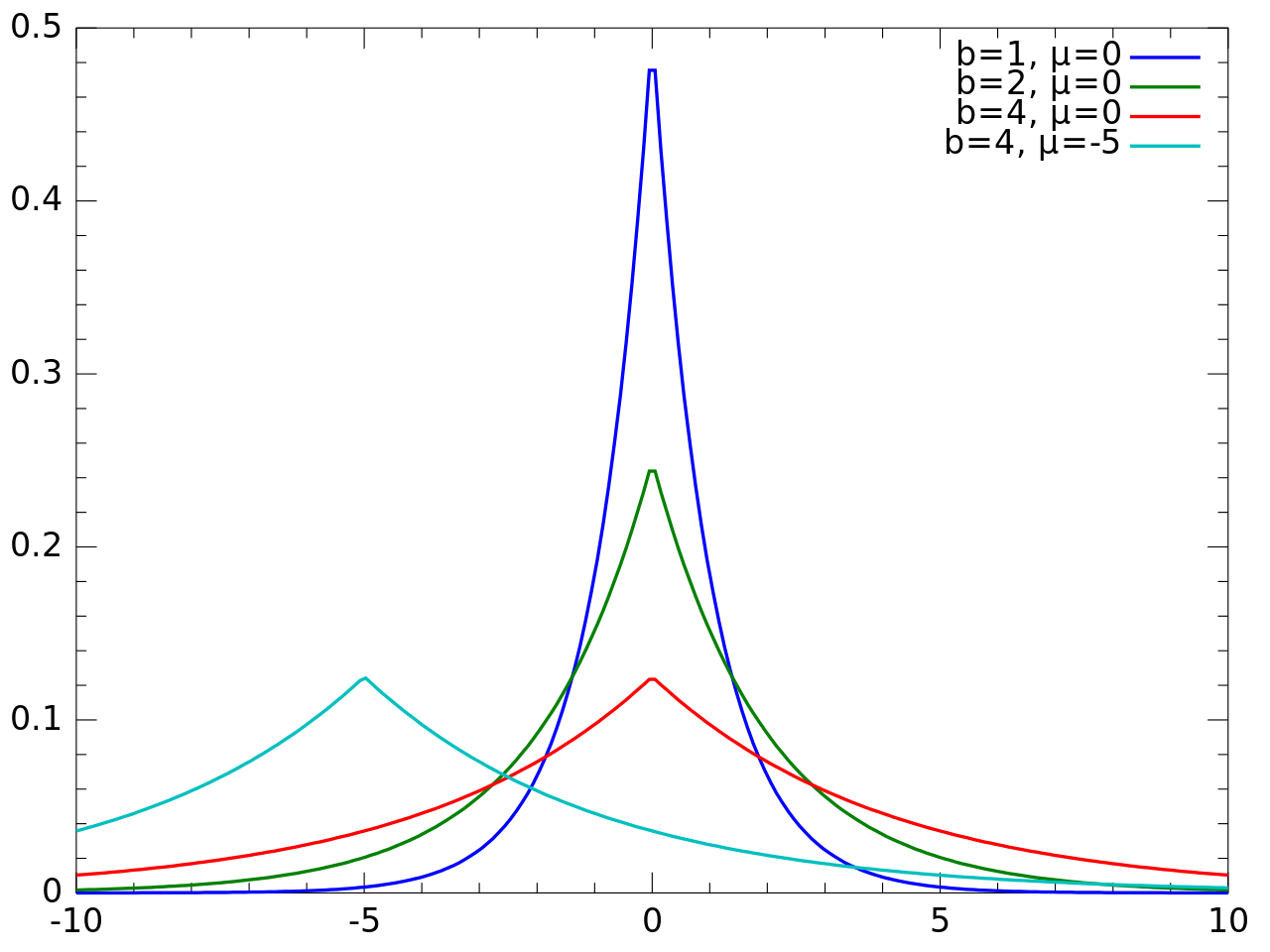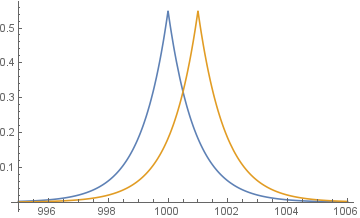Having gone through the importance of differential privacy and its definition, this article motivates the theory with a practical example to make it more intuitive how the definition is applied to obtain privacy guarantees. The small town example in this previous article is just to develop an intuitive understanding of why DP would be important. In practice, for DP to be effective you would need a really large dataset such that the law of large numbers work. The law of large numbers is a law from statistics which proves that even in presence of some noisy measurements, the result of a large number of samples would converge to the true value (or very close to).

Consider the query release problem where a analyst is querying a dataset with the two queries resulting in dataset without a given row (D2) and database with the given row(D1) as defined in previous tutorial.The previous tutorial defined DP as two probability distributions being close to each other by a factor of exp(epsilon). This definition is put into practice by adding noise to result of every query. The noise is sampled from a probability distribution that is calibrated according to the definition of DP. Note that the noise is added to the result of a query and not to the data itself. Since DP defines how two probability distributions vary by a factor of epsilon , we are free to sample from any probability distribution. Most popular distributions used in practice are Laplace and Gaussain.

Note: The article provides a high level overview and intuitive understanding of various mechanisms. For more comphrehensive proofs and details check out the book Algorithmic Foundations of Differential Privacy

## Laplace MechanismLaplace mechanism is a DP mechanism in which noise sampled from a Laplacian distribution is added to a query result. The Laplace distribution is very suitable for DP because of its sharp rise and fall for a given standard deviation.

Laplace distribution is given bydenoted by Lap(μ,b), where μ is the mean of the distribution and b is the standard deviation or scale. The Laplace noise we add is given by Lap(0,sensitivity/epsilon). This gives a privacy guarantee of DP(epsilon,0).

Below is an example of adding Laplacian noise to a counting query. A counting query is a type of query where you find the number of occurances or counts of a given data. Given that the true value is 1001. Consider two queries, the true value D1 with count 1001 and the value in which a count is dropped 1000(D2). We add Laplacian noise to both the datasets.Notice that when sampled from a probability distribution, when we query with just dataset D1 and D2 if the noise added is very close at both instances, there is very little privacy guarantee. But, if both the noise are in opposite direction there is a maximal privacy guarantee. So the probability of the noises added depends on the standard deviation of the distribution we sample from. Based on the above we could develop a mechanism in which we sample for D1 and always add noise in opposite direction when D2 is queried, but we want to develop a generalized querying mechanism where we treat even differencing attacks query as the same as any other query. The adversery having a knowledge of few rows in the database wont find it hard to find out such a mechanism. Hence we rely on DP being a randomized mechanism rather than adding a fixed noise to every result of a query.